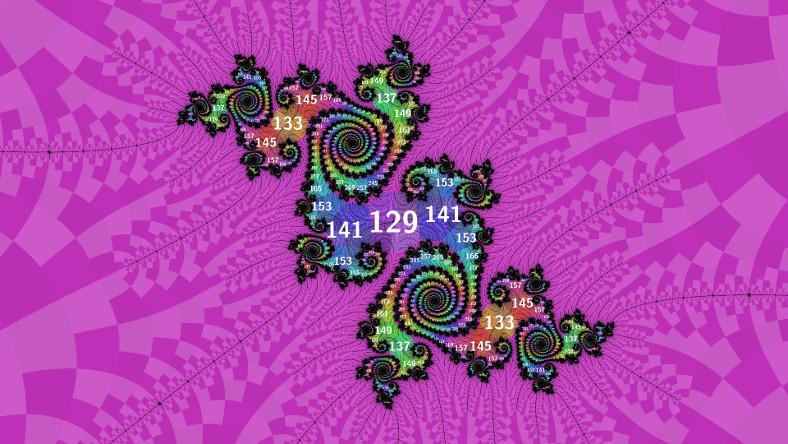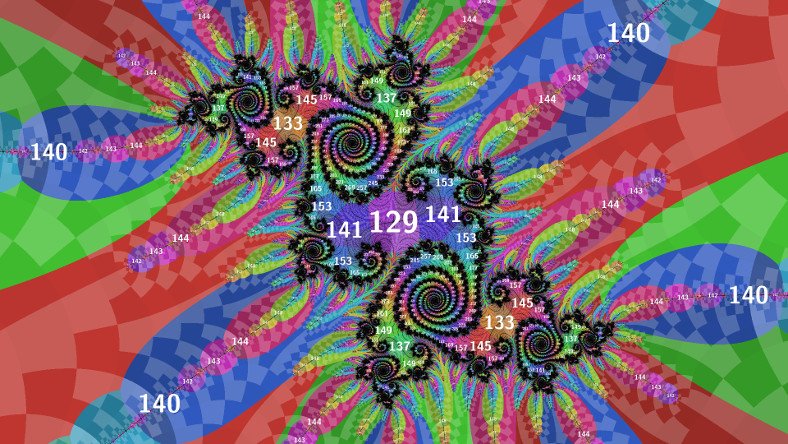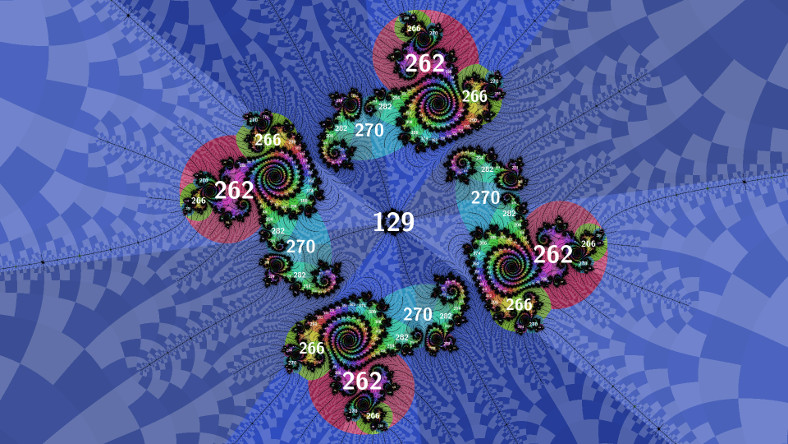# mathr / blog / #

## Filtered atom domainsAbove is what atom domain rendering typicaly looks like. But there is a wealth of information in the periods of islands in the filaments, which isn't visible unless you scan for periods and annotate the image with labels (as in my previous post). After some experimentation, I figured out a way to make their domains visible and moreover get a domain size estimate (useful for labelling).The hack I came up with is in two parts: the first part is in the iteration calculations. Atom domain calculation typically works like this:

for (iteration = 1; iterating; ++iteration)
{
z = z * z + c;
if (abs(z) < minimum)
{
minimum = abs(z);
p = iteration;
zp = z;
}
...
}

The filtering hack adds another pair of $$(q, z_q)$$ to the existing $$(p, z_p)$$, only this time filtered by a function of iteration number:

  ...
if (abs(z) < minimum2 && accept(iteration))
{
minimum2 = abs(z);
q = iteration;
zq = z;
}
...

For the image above the accept() filter function was:

bool accept(int p)
{
return p >= 129 && (p % 4) != 1;
}

The second part of the hack is filtering when colouring, to allow the original regular atom domains to be visible too (without this part they get squashed by the new domains). Here's the colouring hack for the image above:

  ...
int p = (computed p);
double _Complex zp = (computed zp);
if (reject(p))
{
p = (computed q);
zp = (computed zq);
}
// colour using p and zp
...

bool reject(int p)
{
return p < 129;
}

The image below uses slightly different filters:

bool accept(int p)
{
return p >  129 && (p % 4) == 2;
}

bool reject(int p)
{
return p <= 129 || (p % 4) == 1;
}I did manage to find some filters that showed domains in the filaments at this deeper zoom level, but I lost them while making other changes and I've been unable to recreate them - very frustrating.

These images show another minor development, colouring the domains according to the quadrants of $$z_p$$, which meet at the nucleus. I was inspired to do this by Algorithm 9 of Wolf Jung's Mandel which shows the zeros of a particular period (I wanted to show all periods at once). This forms the basis of an improved periodicity scan algorithm, which iterates an image one step at a time, scanning for meeting quadrants at a local minimum of $$z_p$$ and recording their locations and periods to an output buffer. This new algorithm is much more scalable to deep zooms (it will work fine with perturbation techniques, though I haven't implemented that yet - the previous algorithm definitely needed lots of arbitrary precision calculations). It might even be possible to accelerate on GPU, its parallelism is amenable.

The size estimate for the filtered domains is fairly similar to the atom domain size estimate I derived previously:

double filtered_domain_size(double _Complex nucleus, int period)
{
double _Complex z = 0;
double _Complex dc = 0;
double zq = 1.0/0.0;
for (int q = 1; q <= period; ++q)
{
dc = 2 * z * dc + 1;
z = z * z + nucleus;
double zp = cabs(z);
if (q < period && zp < zq && accept(q))
{
zq = zp;
}
}
return zq / cabs(dc);
}

This can return infinity if the filter is too restrictive, but domain size of the period 1 cardioid is infinite too, so it's not a big deal for the caller to check and deal with it as appropriate.

I'll clean up the code a bit and push to my mandelbrot-* repositories soon.